﻿ 天波雷达后多普勒自适应波束形成
«上一篇文章快速检索 高级检索

 雷达学报2016, Vol. 5Issue (4): 373-377  DOI: 10.12000/JR151240

### 引用本文 [复制中英文]

[复制中文]
Chen Xixin . Post-Doppler Adaptive Digital Beamforming of Skywave Radar[J]. Journal of Radars, 2016, 5(4): 373-377. DOI: 10.12000/JR15124.
[复制英文]

### 文章历史

(中国电子科技集团公司第十四研究所 南京 210039)

Chen Xixin(The No.14 Institute of CETC, Nanjing 210039, China)
Foundation Item: The National Natural Science Foundation of China (61271327, 61071164)
Abstract: The analytical expression of shortwave interference in the range-Doppler domain is derived and is found to exhibit a constant-amplitude spectrum ridge parallel to the range axis. The spatial nonstationarity of the shortwave interference induced by ionosphere perturbation is then analyzed and is found to be equivalent to the amplitude-phase error between the same frequency points of shortwave interference on different antenna elements; hence, the above mentioned spatial nonstationarity only a has a slight effect on the performance of Adaptive Digital BeamForming (ADBF). On the basis of the above analyses, this paper presents a post-Doppler ADBF approach for skywave radar. This approach involves transforming the received signal in each antenna element into a range-Doppler domain and then performing adaptive processing at each Doppler frequency point. The real radar data processing conducted in this study shows that the ADBF approach has a good interference suppression performance and strong robustness.
1 引言

2 雷达信号模型与短波干扰特性 2.1 雷达信号模型

 $a\left( \theta \right)=\left[ 1\exp \left( j2\pi /\lambda d\sin \left( \theta \right) \right)\cdots \exp \left( j2\pi /\lambda \left( N-1 \right)d\sin \left( \theta \right) \right) \right]$

 $x(m,t)=s(m,t)+c(m,t)+z(m,t)+n(m,t),m=1,\cdots ,M$ (1)

2.2 短波干扰的距离-多普勒谱

OTHR发射线性调频连续波信号，表示为：

 $u(t)=\text{rect}\left( t/{{T}_{\text{r}}} \right)\exp (\text{j}\pi K{{t}^{2}})\$ (2)

 ${{v}_{m}}(t)=\text{rect}\left( \frac{t-m{{T}_{\text{r}}}}{{{T}_{\text{r}}}} \right)\exp (\text{j2}\pi {{f}_{1}}t),-B/2<{{f}_{1}} 当BT$\gg $1时，匹配滤波器的近似频率响应为 $G(f)=\text{rect}\left( \frac{f}{B} \right)\exp \left( \text{j}\pi \frac{{{f}^{2}}}{K} \right)\ $(4) 该滤波器的群延迟为： ${{t}_{\text{d}}}(f)=-\frac{f}{K}$(5) 因此短波干扰通过匹配滤波器后的输出为： $\begin{align} & {{y}_{m}}(t)={{v}_{m}}[t-{{t}_{\text{d}}}({{f}_{1}})]=\text{rect}\left( \frac{t+{{f}_{1}}/K-m{{T}_{\text{r}}}}{{{T}_{\text{r}}}} \right) \\ & \cdot \exp [\text{j2}\pi {{f}_{1}}(t+{{f}_{1}}/K)] \\ \end{align}$(6) t'=t - mTr，则式(6)变为： $\begin{align} & {{y}_{m}}({t}')=\text{rect}\left( \frac{{t}'+{{f}_{1}}/K}{{{T}_{\text{r}}}} \right) \\ & \cdot \exp [\text{j2}\pi {{f}_{1}}({t}'+{{f}_{1}}/K+m{{T}_{\text{r}}})] \\ \end{align}$(7) 式(7)表明短波干扰可以无失真地通过匹配滤波器，分布于全程距离上。 在对各重复周期上的接收信号进行脉冲压缩处理后，OTHR还要对各距离单元上的接收信号进行相干积累处理。由式(7)可见，短波干扰也会同样实现相干积累，若有M个连续的相干脉冲，则可以得到： $\begin{align} & Y({t}',{{f}_{\text{d}}})=M\cdot \exp [\text{j2}\pi {{f}_{1}}({t}'+{{f}_{1}}/K)+\text{j}\pi ({{f}_{1}}-{{f}_{\text{d}}}) \\ & \cdot (M-1){{T}_{\text{r}}}]\cdot \text{sinc}\pi ({{f}_{1}}-{{f}_{\text{d}}})M{{T}_{\text{r}}}] \\ \end{align}$(8) 式(7)和(8)表明，短波干扰在距离−多普勒谱图上表现为平行于距离轴的恒幅谱脊，其位置为${f_{{\rm{d1}}}}=\bmod ({f_1},\;{f_{\rm{r}}}),{f_{\rm{r}}}=1/{T_{\rm{r}}}$为脉冲重复频率。前面为了推导的方便假设短波干扰是单频的，实际短波干扰总有一定的带宽，会造成谱脊位置抖动和基底抬高，但是基本特征不会改变。 2.3 短波干扰的空间非平稳性 当短波干扰通过电离层反射进入OTHR接收机时，由于电离层是运动的反射媒介，经它反射的短波干扰通常是空间非平稳的，即干扰的角度随时间变化。 在脉冲压缩后，第n个阵元、第m个脉冲、第k个距离门上的干扰表示为： $\begin{align} & z(n,m,k)=\exp \left[ \text{j}\frac{2\pi }{\lambda }nd\sin ({{\theta }_{m}}) \right] \\ & \cdot {{y}_{m}}(\text{ }t_{k}^{\prime }),\ n=0,1,\cdots ,N-1 \\ \end{align}$(9) 式中θm为干扰的方位角，这里假设它是慢时变的，即在脉冲重复周期之间变化，而在1个脉冲重复周期内不变化。 设短波干扰的初始方位角为θ，有θm=θθm，若δθm较小，则式(9)可近似表示为： $\begin{align} & z(n,m,k)\approx \exp \left[ \text{j}\frac{2\pi }{\lambda }nd\sin (\bar{\theta }) \right] \\ & \cdot \exp \left[ \text{j}\frac{2\pi }{\pi }nd\cos (\bar{\theta })\cdot \delta {{\theta }_{m}} \right]\cdot {{y}_{m}}(\text{ }t_{k}^{\prime }) \\ \end{align}$(10) 式(10)右边的第2项刻画了干扰的空间非平稳性，与阵元n和角度变化量δθm有关。 将式(10)变换到多普勒域上得到： $\begin{align} & Z(n,{{f}_{\text{d}}},k)\approx \exp \left[ \text{j}\frac{2\pi }{\lambda }nd\sin (\overline{\theta }) \right] \\ & \cdot \left[ \Gamma (n,{{f}_{\text{d}}})\otimes Y(\text{ }t_{k}^{\prime },{{f}_{\text{d}}}) \right] \\ \end{align}$(11) 式中：Γ(n,fd)是式(10)右边第2项的频谱，Y(t'k,fd)是第3项的频谱，⊗表示卷积运算。 忽略式(8)中辛格函数的副瓣，式(11)中的卷积运算将辛格函数的主瓣搬移到多个多普勒频点上，幅度按Γ(n,fd)分布。对于不同的阵元，频谱Γ(n,fd)是变化的，但是对于单个阵元，它在所有距离单元上都相同。当在各个频点上分别进行ADBF处理时，变化的Γ(n,fd)等效于阵元之间的幅相误差，对ADBF性能的影响可以忽略，因此短波干扰能够被有效地抑制。 3 自适应波束形成器 上一节的分析表明，在距离-多普勒域上，短波干扰沿距离维是平稳的，电离层运动转化为阵元之间的幅相误差，对ADBF性能的影响并不大，另外，在天波雷达的对空探测中，目标信号通常位于非杂波区，因此本文提出在距离-多普勒域上进行自适应处理，即后多普勒自适应波束形成，以抑制非杂波区中的短波干扰。 OTHR接收阵规模庞大，为了降低自适应处理的复杂度，ADBF采用广义副瓣对消结构，如图 1所示，首先将各阵元接收信号分别变换到距离-多普勒域上，然后执行副瓣对消自适应处理，其中上支路实现常规波束形成，下支路计算ADBF权矢量用来对消上支路中的短波干扰，虚线框中给出了第m个多普勒单元上的自适应波束形成。B为阻塞矩阵，满足条件BHa(θ0)=0，从而阻塞了目标信号进入下支路。为了降维，B的列数只要略大于干扰数即可，式(12)是一个N ×(N -1)维的两阵元差波束系数矩阵，从中随机选择若干列构成矩阵B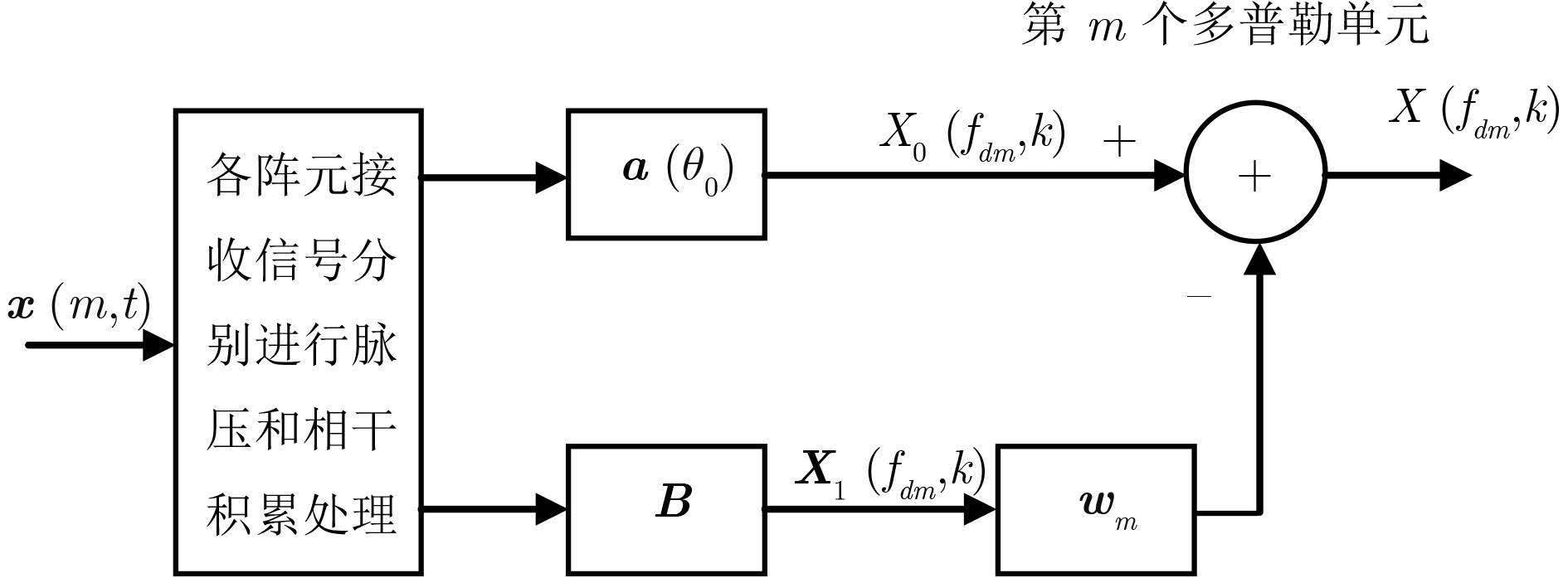图 1 广义副瓣对消器 Fig.1 Generalized sidelobe canceller ${{B}_{0}}=\left[ \begin{matrix} 1 & 0 & \cdots & 0 & 0 \\ b & 1 & \cdots & \vdots & 0 \\ 0 & b & \ddots & 0 & \vdots \\ \vdots & 0 & \ddots & 1 & 0 \\ 0 & \vdots & \cdots & b & 1 \\ 0 & 0 & \cdots & 0 & b \\ \end{matrix} \right]$(12) 式中b=-exp[j2πdsin(θ0)]。 在距离-多普勒域的非杂波区中，ADBF处理按多普勒单元进行，训练样本沿距离维取得。对于第m个单元，设上支路输入信号为X0(fdm,k)，下支路输入信号为X1(fdm,k)，则副瓣对消器输出为： $X({{f}_{\text{d}m}},k)={{X}_{0}}({{f}_{\text{d}m}},k)-\omega _{m}^{\text{H}}{{X}_{1}}({{f}_{\text{d}m}},k)$(13) 式中 ${{w}_{m}}=R_{m}^{-1}{{r}_{m}}$(14) ${{R}_{m}}=\sum\limits_{k}{{{X}_{1}}({{f}_{\text{d}m}},k)X_{1}^{\text{H}}({{f}_{\text{d}m}},k)}$(15) ${{r}_{m}}=\sum\limits_{k}{{{X}_{1}}({{f}_{\text{d}m}},k)\text{ }X_{0}^{*}({{f}_{\text{d}m}},k)}\$ (16)
4 实测数据处理与分析 4.1 无杂波数据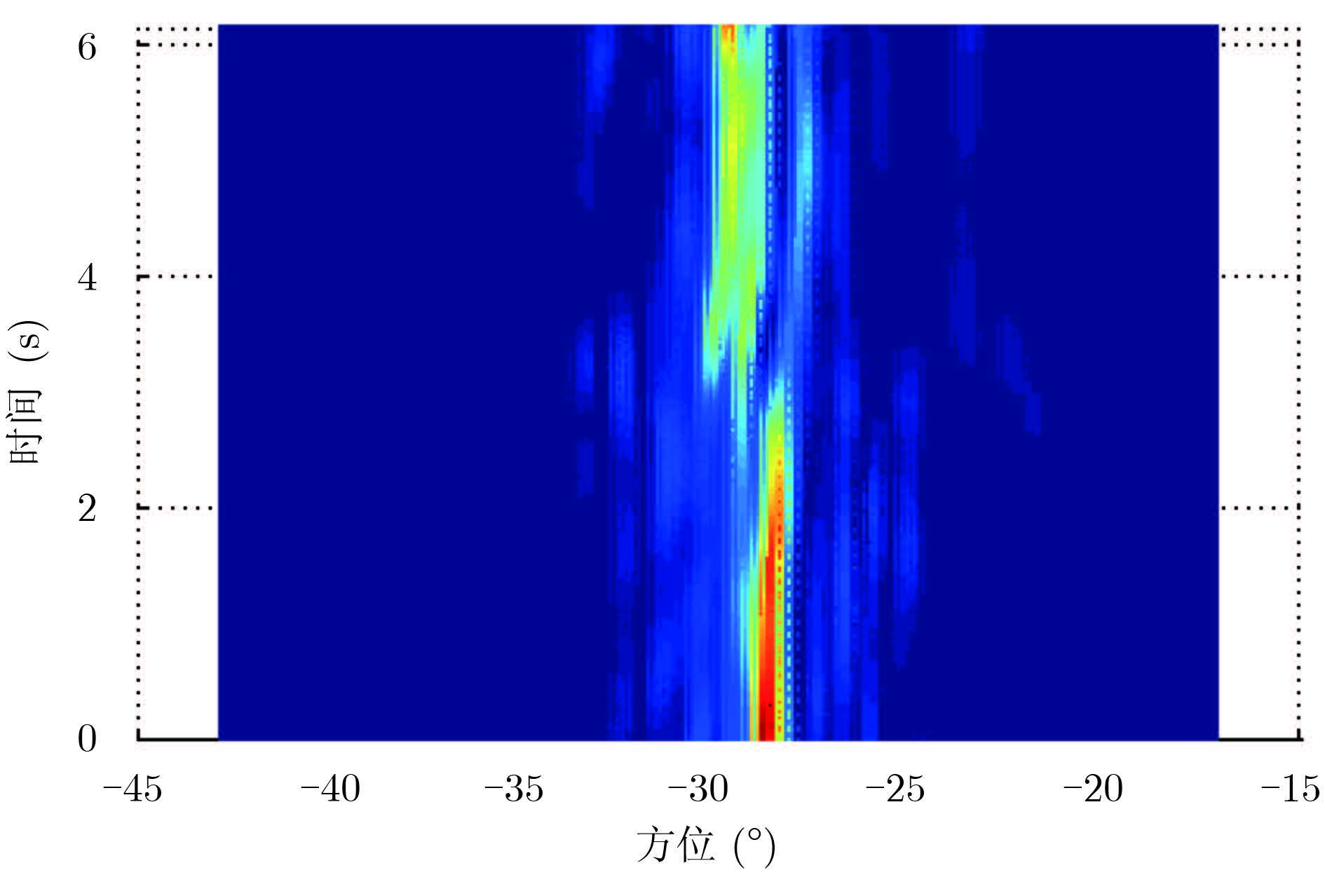图 2 干扰的MUSIC谱 Fig.2 MUSIC spectrum of interference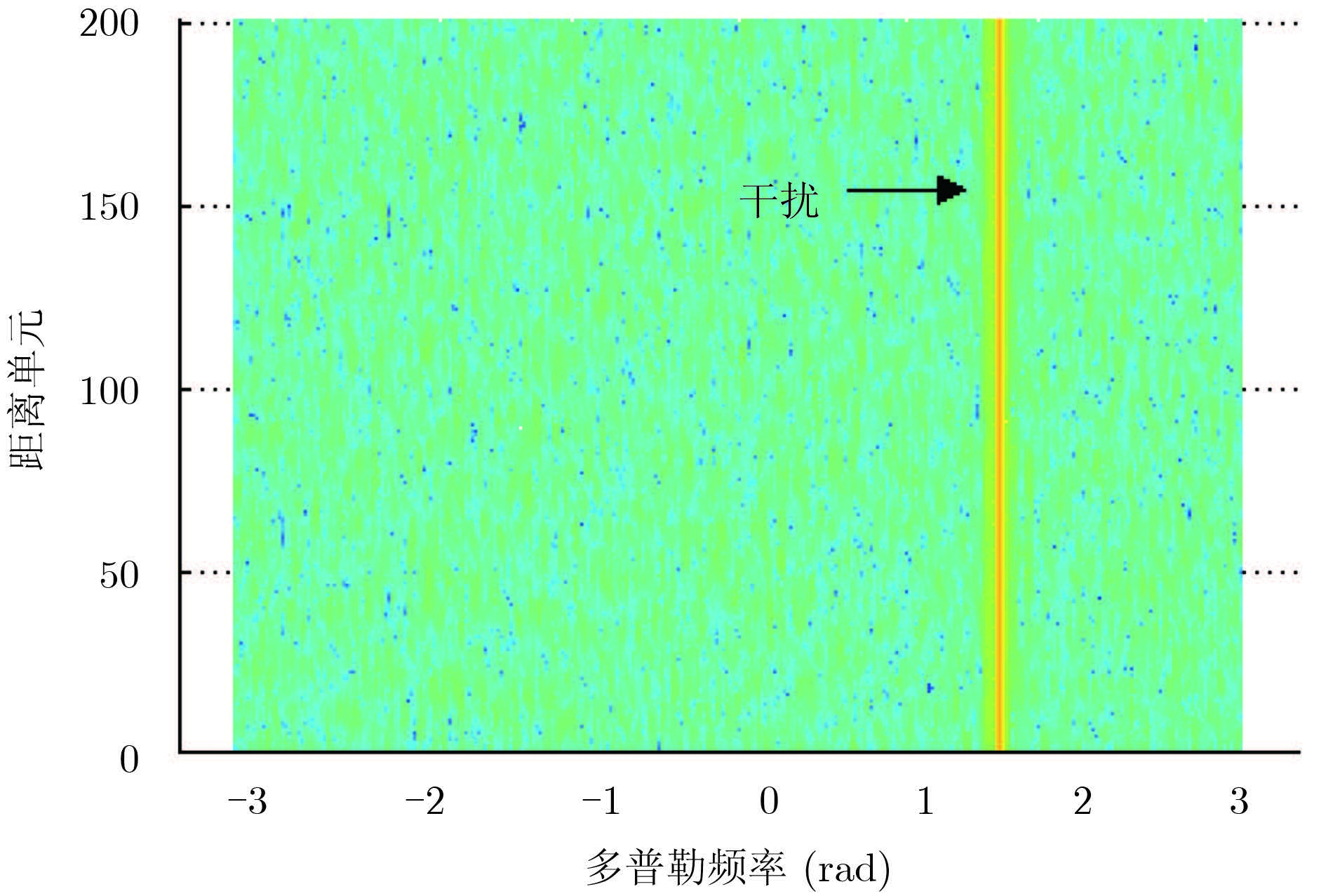图 3 干扰的距离-多普勒谱 Fig.3 Range-Doppler spectrum of interference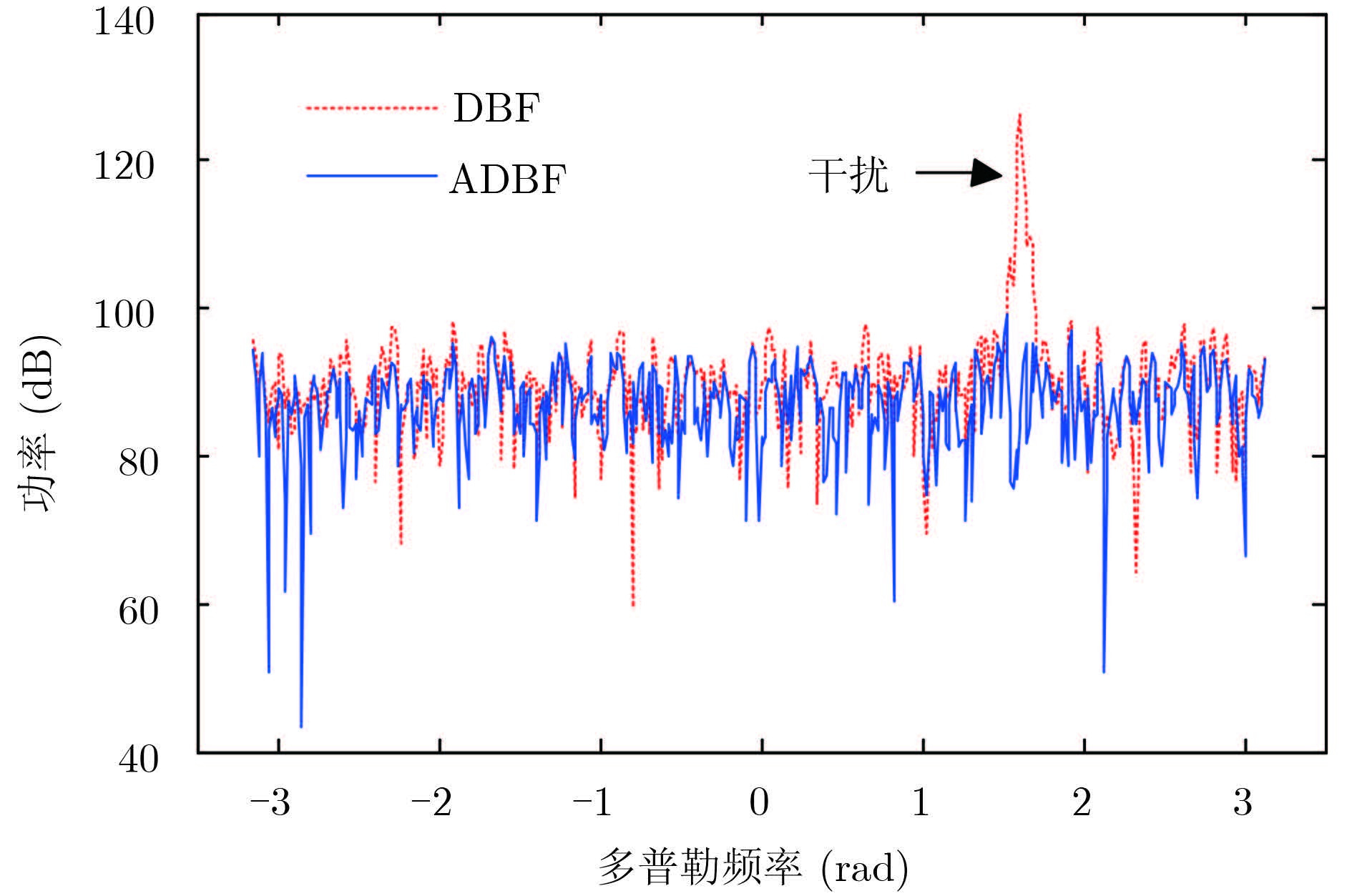图 4 DBF与ADBF的比较 Fig.4 Comparison of DBF and ADBF
4.2 有杂波数据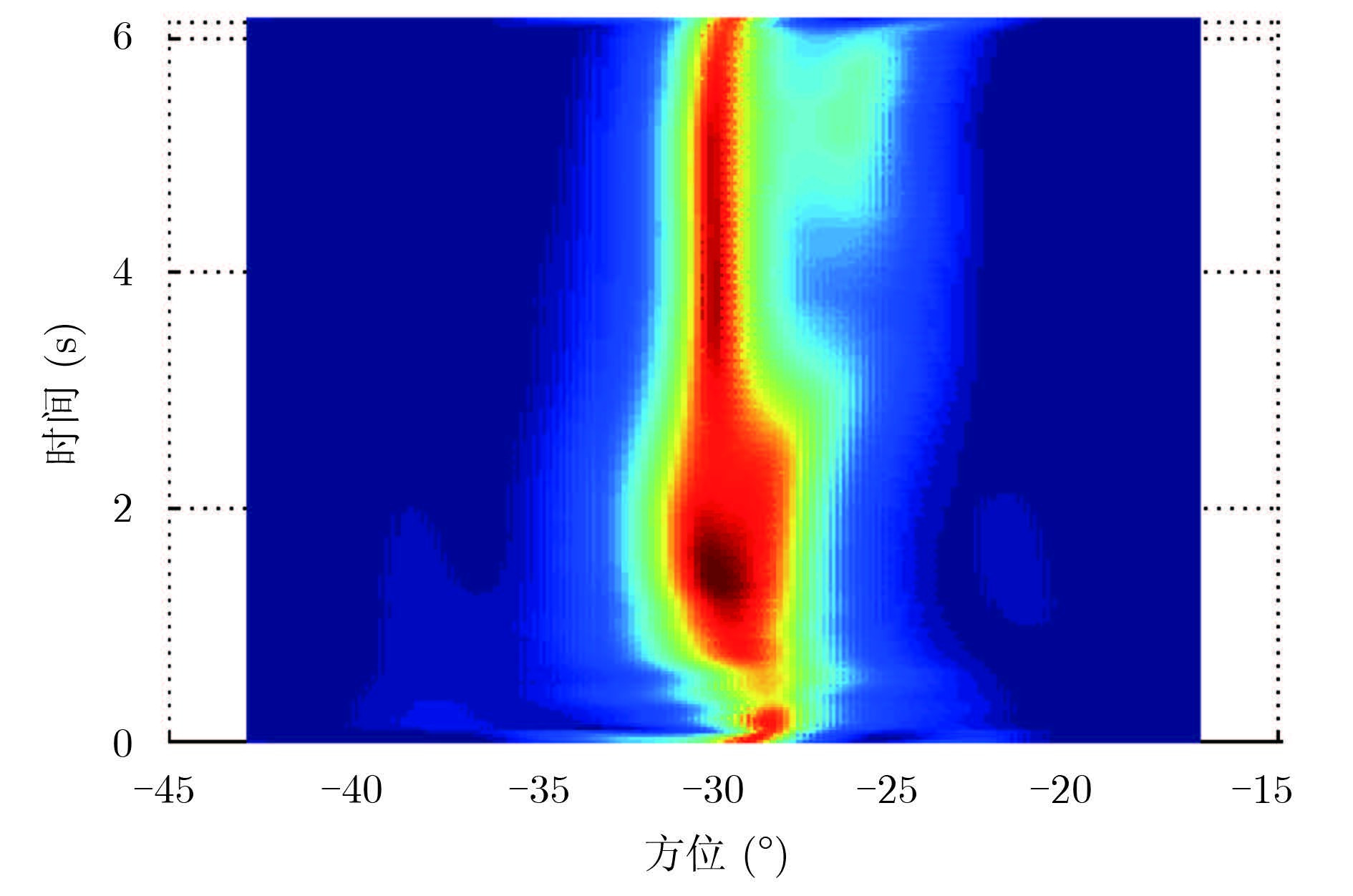图 5 干扰1的MUSIC谱 Fig.5 MUSIC spectrum of interference 1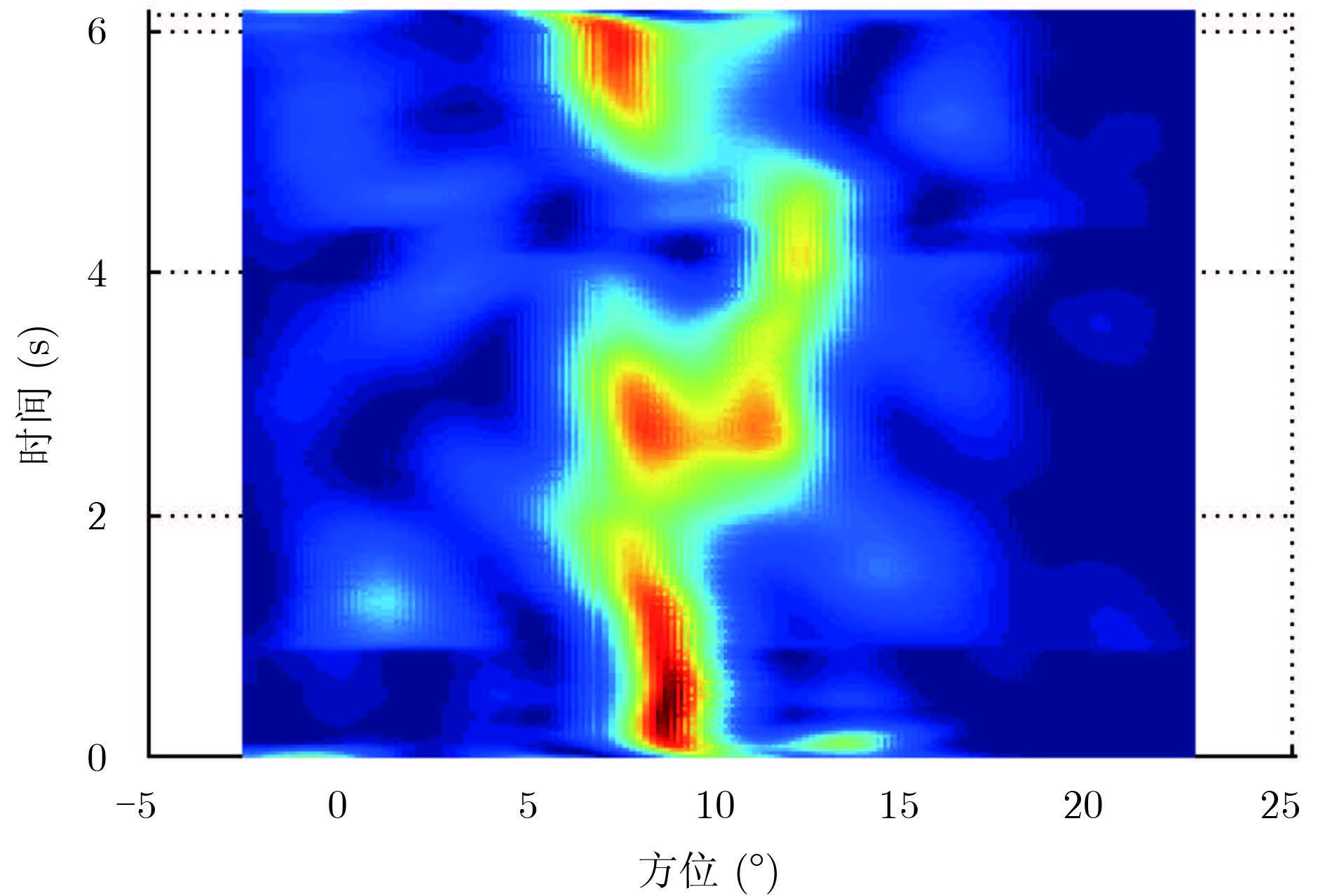图 6 干扰2的MUSIC谱 Fig.6 MUSIC spectrum of interference 2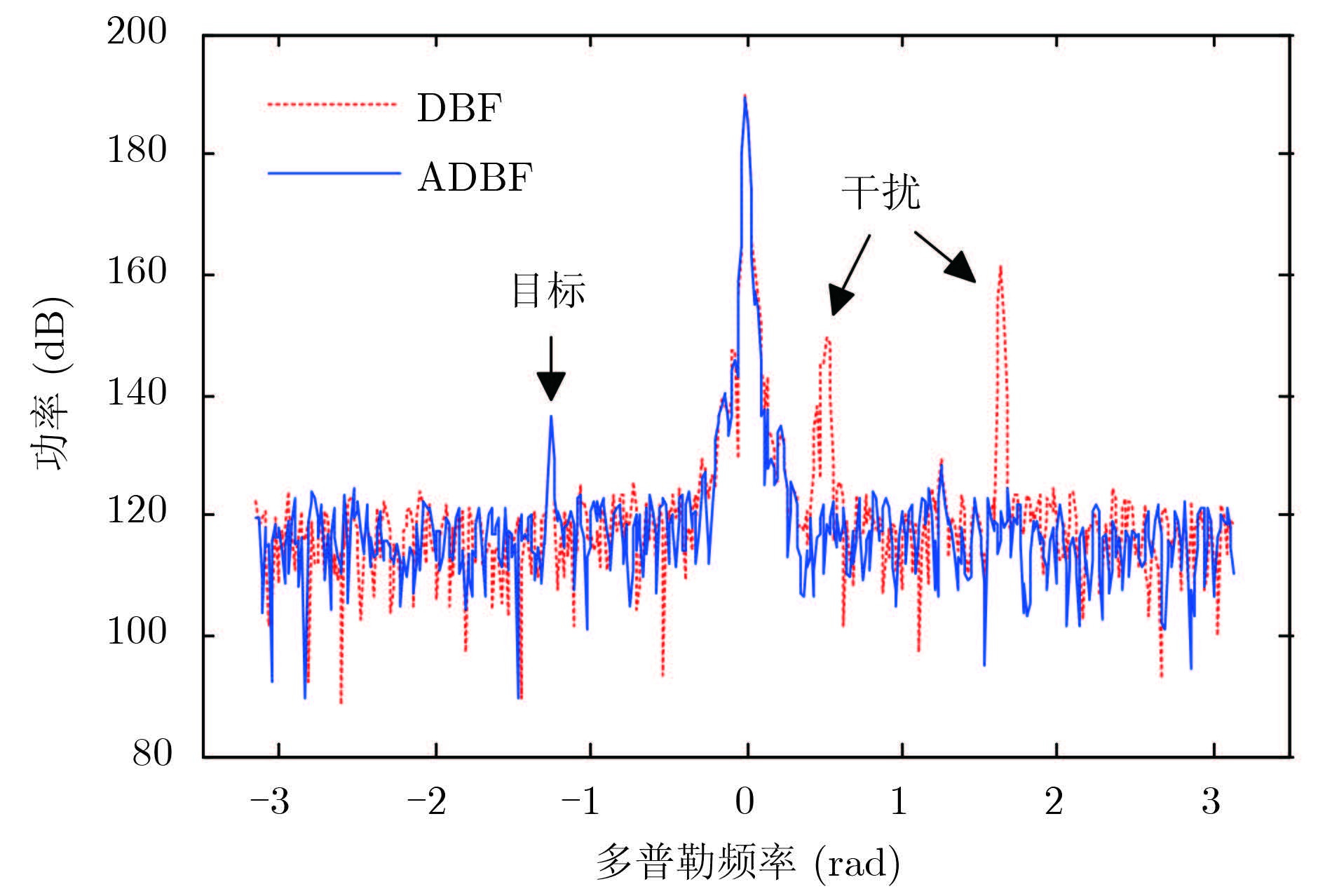图 7 DBF与ADBF的比较 Fig.7 Comparison of DBF and ADBF
  周文瑜, 焦培南. 超视距雷达技术[M]. 北京: 电子工业出版社, 2008 : 235 -243. Zhou Wen-yu, Jiao Pei-nan. Over-The-Horizon Radar Technique[M]. Beijing: Publishing House of Electronics Industry, 2008 : 235 -243. (0)  Fabrizio G A, Abramovich Y I, Anderson S J. Adaptive cancellation of nonstationary interference in HF antenna array[J]. IEE Proceedings-Radar, Sonar and Navigation,1998, 145 (1) : 19 –24. (0)  唐晓东, 周文瑜. 短波雷达干扰与抗干扰技术[J]. 现代雷达,2003, 25 (2) : 21 –25. Tang Xiao-dong, Zhou Wen-yu. Interference and anti-interference techniques of HF radar[J]. Modern Radar,2003, 25 (2) : 21 –25. (0)  沈石坚, 黄银和, 朱炳元. 高频雷达干扰特性研究[J]. 现代雷达,2008, 30 (11) : 30 –33. Shen Shi-jian, Huang Yin-he, Zhu Bing-yuan. A study on interference characteristics in high frequency radar[J]. Modern Radar,2008, 30 (11) : 30 –33. (0)  Fabrizio G A, Gershman A B, Turley M D. Robust adaptive beamforming for HF surface wave radar[J]. IEEE Transactions on Aerospace and Electronic Systems,2004, 40 (2) : 510 –524. (0)  李高鹏, 李雷, 许荣庆. 基于海杂波保持的高频地波雷达干扰抑制方法研究[J]. 电波科学学报,2005, 20 (3) : 358 –362. Li Gao-peng, Li Lei, Xu Rong-qing. RF interference suppression method based on sea-clutter-wave maintaining for HF ground-wave radar[J]. Chinese Journal of Radio Science,2005, 20 (3) : 358 –362. (0)  Su H T, Liu H, Shui P, et al. Adaptive HF interference cancellation for sky wave over-the-horizon radar[J]. Electronics Letters,2011, 47 (1) : 50 –52. (0)  陈希信, 韩彦明, 于景兰. 高频雷达自适应波束形成抗干扰研究[J]. 电波科学学报,2010, 25 (6) : 1169 –1174. Chen Xi-xin, Han Yan-ming, Yu Jing-lan. Research for interference suppression in HF radar based on ADBF[J]. Chinese Journal of Radio Science,2010, 25 (6) : 1169 –1174. (0)  周浩, 文必洋, 吴世才, 等. 应用时频分析进行高频雷达射频干扰抑制[J]. 电子学报,2004, 32 (9) : 1546 –1548. Zhou Hao, Wen Bi-yang, Wu Shi-cai, et al. HF radar radio frequency interference suppression based on time-frequency analysis[J]. Acta Electronica Sinica,2004, 32 (9) : 1546 –1548. (0)  林茂庸, 柯有安. 雷达信号理论[M]. 北京: 国防工业出版社, 1984 : 133 -134. Lin Mao-yong and Ke You-an, Ke You-an, Ke You-an. Radar Signal Theory[M]. Beijing: National Defense Industry Press, 1984 : 133 -134. (0)  王永良, 丁前军, 李荣峰. 自适应阵列处理[M]. 北京: 清华大学出版社, 2009 : 276 -282. Wang Yong-liang, Ding Qian-jun, Li Rong-feng. Adaptive Array Processing[M]. Beijing: Tsinghua University Press, 2009 : 276 -282. (0)